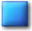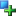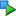##Image_Graph_Data_Bar

Description

Bar data-element

This class is used to draw a bar in the diagram. Support for stacking is implemented in this element.

Located in /Graph/Data/Bar.php (line 45)

```Image_Graph_Data_Common
|
--Image_Graph_Data_Bar```
Method SummaryImage_Graph_Data_Bar Image_Graph_Data_Bar (object Image_Graph &\$parent, array \$data, [array \$attributes = array()])void drawGD (resource &\$img, [int \$drawWhat = IMAGE_GRAPH_DRAW_FILLANDBORDER])void stackingPrepare (array &\$dataElements)
MethodsConstructor Image_Graph_Data_Bar (line 55)

Constructor

• access: public
Image_Graph_Data_Bar Image_Graph_Data_Bar (object Image_Graph &\$parent, array \$data, [array \$attributes = array()])
• object Image_Graph &\$parent: parent object
• array \$data: numerical data to be drawn
• array \$attributes: attributes like colordrawGD (line 120)

Draws the data element, using GD-output

The parameter \$drawWhat is needed to be able to first draw the fill of all bar-elements and later draw the border, in case that stacking is used.

• access: public
void drawGD (resource &\$img, [int \$drawWhat = IMAGE_GRAPH_DRAW_FILLANDBORDER])
• resource &\$img: GD-resource to draw to
• int \$drawWhat: choose what to draw; use constants IMAGE_GRAPH_DRAW_FILLANDBORDER, IMAGE_GRAPH_DRAW_JUSTFILL or IMAGE_GRAPH_DRAW_JUSTBORDER

Redefinition of:
Image_Graph_Data_Common::drawGD()
Draws diagram elementstackingPrepare (line 74)

Prepare given dataElements of this type for stacking

• static:
• access: public
void stackingPrepare (array &\$dataElements)
• array &\$dataElements: references to dataElements (objects of this type)

#### Inherited Methods

Inherited From Image_Graph_Data_CommonImage_Graph_Data_Common::Image_Graph_Data_Common()Image_Graph_Data_Common::drawGD()Image_Graph_Data_Common::setColor()Image_Graph_Data_Common::setDataMarker()Image_Graph_Data_Common::setFill()Image_Graph_Data_Common::stackingDrawGD()

Documentation generated on Sat, 21 Feb 2004 23:28:00 +0100 by phpDocumentor 1.3.0RC2